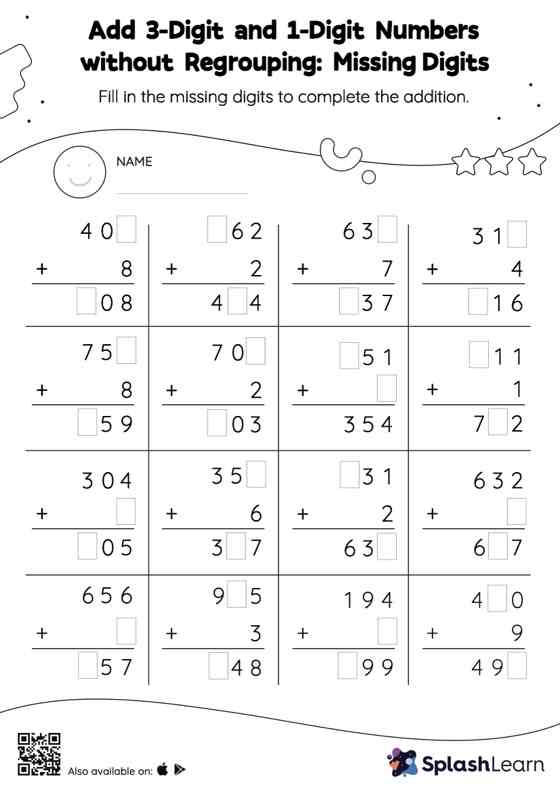# Add 3-Digit and 1-Digit Numbers without Regrouping: Missing Digits Worksheet

Home > Add 3-Digit and 1-Digit Numbers without Regrouping: Missing DigitsThis worksheet challenges young mathematicians to hone their math skills by solving a set of problems on adding 3-digit and 1-digit numbers without regrouping. In this worksheet, students must find the missing number using the count-on method or the relationship between addition and subtraction. To solve add 3-digit and 1-digit numbers without regrouping worksheet, they do not need to regroup the numbers. This worksheet is about practicing the column method in which numbers are written one on top of another. In this method, students use their place value understanding to solve the problems.